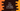# C++ program to count total digits in a number# Introduction :

In this tutorial, we will learn how to count the total digits in a number in C++. The program will ask the user to enter the number. It will count the digits and print it out. We will learn different ways to solve this problem.

## Method 1: Using a loop :

If we divide one integer by 10, it’s rightmost digit is removed. e.g. 122/10 is 12. We can keep dividing the number until it becomes 0. For example, we can divide 1234 four times until it becomes 0. Using a loop, the program will keep dividing the number by 10 and one variable will keep the count.

The final C++ program is :

``````#include <iostream>
using namespace std;

int main()
{
//1
int number;
int total = 0;

//2
cout << "Enter a number : " << endl; cin >> number;

//3
while (number != 0)
{
total++;
number = number / 10;
}

//4
cout << "Total digits in the number : " << total << endl;

return 0;
}``````

### Explanation :

The commented numbers in the above program denote the step numbers below :

1. Create two integer variables. One to store the user-provided number and the other to store the total digit count.
2. Ask the user to enter a number. Read and store it in a variable number.
3. Run one while loop. This loop will run until the value of number becomes 0. On each iteration of the loop, increment the value of total by 1 and change the value of number to number/10.
4. Finally, once the loop is completed, print the total digits count i.e. the value of total.

### Sample output :

``````Enter a number :
12567
Total digits in the number : 5``````

## Recursive method :

We can also calculate the total count recursively. In a recursive approach, the same method is called again and again.

``````#include <iostream>
using namespace std;

int findTotalCount(int number)
{
if (number == 0)
{
return 0;
}
return 1 + findTotalCount(number / 10);
}

int main()
{
int number;

cout << "Enter a number : " << endl; cin >> number;

cout << "Total digits in the number : " << findTotalCount(number) << endl;

return 0;
}``````

Here, we are calling the findTotalCount method recursively. It takes one parameter number. If the value of number is 0, it returns 0. Else, it calls the same method and increases the current count by 1.

It will produce the same output as the previous example.

## By converting to a string :

This is a quick and easy method. C++ string comes with one inbuilt method to find the length. Also, we can convert an integer to a string using the tostring_ method provides in string header. That’s it. Just convert the number to a string and find out the length of that string :

``````#include <iostream>
#include <string>
using namespace std;

int main()
{
int number;

cout << "Enter a number : " << endl; cin >> number;

cout << "Total digits in the number : " << to_string(number).size()<< endl;

return 0;
}``````

It will print the same output.

## Using log :

Another quick way to find out the total digits. We can find out the round of log10 value of the number and add 1 to it to find out the count :

``````#include <iostream>
#include <cmath>
using namespace std;

int main()
{
int number;

cout << "Enter a number : " << endl; cin >> number;

cout << "Total digits in the number : " << 1 + floor(log10(number)) << endl;

return 0;
}``````